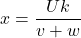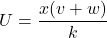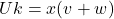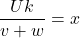U=x(v + w)/k solve for x Helpppppp

Question

U=x(v + w)/k solve for x
Helpppppp

in progress 0
5 months 2021-08-16T05:04:31+00:00 1 Answers 3 views 0General Formulas and Concepts:

Pre-Algebra

Order of Operations: BPEMDAS

1. Brackets
2. Parenthesis
3. Exponents
4. Multiplication
5. Division
7. Subtraction
• Left to Right

Equality Properties

• Multiplication Property of Equality
• Division Property of Equality1. [Multiplication Property of Equality] Multiply k on both sides:2. [Division Property of Equality] Isolate x:3. Rewrite: